﻿ 基于Hvorslev和CBP模型的微水试验应用

# 基于Hvorslev和CBP模型的微水试验应用Application of Slug-Water Test Based on Hvorslev and CBP Model

Abstract: Combined with a project example, the slug-water test is carried out in the low-permeability stratum, and the test results are compared with the traditional packer permeability test results. The results show that the slug-water test results are larger than the packer permeability test. The method is affected by temperature, pore storage effect and skin effect, and the required parameters can only reflect the permeability of the aquifer in the limited radius near the borehole. However, the slug-water test method has the advantages of short test period, low cost, simple equipment, stratified test and no pollution. It has a good prospect in engineering survey.

1. 引言

2. 原理简介

2.1. CBP模型基本原理

CBP模型是基于传导方程确定含水层参数的微水试验模型 ，模型基本假定：承压含水层是均质、各向同性；含水层等厚、无限延伸；承压井为完整井；在井孔工作段的井壁上或滤管的壁面上，任何时候含水层中的水头与井中水位度都是相等的；基准面取在含水层初始水头而上；通过试验井壁流入含水层的流量等于井中水体减少的速率(图1)。在上述假设条件下，可以得到井流问题的数学模型：

1) 水流运动方程

$\alpha \frac{{\partial }^{2}\omega }{\partial {r}^{2}}+\frac{1}{r}\frac{\partial \omega }{\partial r}=\frac{\partial \omega }{\partial t}$ (1)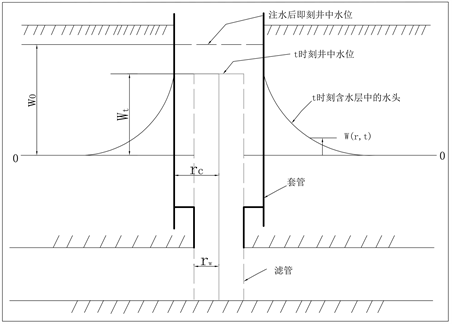Figure 1. Schematic diagram of the CBP model

$2\text{π}{r}_{w}T\frac{\partial \omega \left({r}_{w},t\right)}{\partial t}=\text{π}{r}_{c}^{2}\frac{\partial \omega \left(t\right)}{\partial t}$

2) Cooper等(1967)导出水头变化关系式：

$\omega ={\omega }_{0}F\left(\alpha ,\beta \right)$

$F\left(\alpha ,\beta \right)=\frac{8\alpha }{{\text{π}}^{2}}\underset{0}{\overset{\infty }{\int }}\frac{\mathrm{exp}\left(-\beta {u}^{2}/\alpha \right)}{uf\left(u,\alpha \right)}$ (2)

$\frac{Tt}{{r}_{c}^{2}}=1$ ，记录 $\omega /{\omega }_{0}~t$ 曲线这点对应t的时间，同时记录相应标准曲线的 $\alpha$ 值，则可以求得这些水文地质参数。

$T=\frac{\beta {r}_{c}^{2}}{t};S=\frac{\alpha {r}_{c}^{2}}{{r}_{w}^{2}}$

2.2. Hvorslev模型原理

Hvorslev在1951年通过大量试验后发现井内水位迅速变化后水位恢复的速度和时间成指数关系，水位恢复的时间与地层的渗透系数有关，恢复速率与井孔的结构有关。在此基础上，Hvorslev针对承压完整和非完整井的过阻尼微水试验提出一种半解析的方法 。

$q\left(t\right)=\text{π}{r}_{c}^{2}\frac{\text{d}y}{\text{d}t}=F{K}_{c}\left({h}_{0}-y\right)$ (3)

F：形状因子，取决于井孔滤水管形状和位置；

${K}_{c}$ ：影响半径内的含水层水平渗透系数；

${h}_{0}$ ：t = 0时静止水位到井孔水位间的距离；

y：试验过程井孔水位的变化量；

${h}_{0}-y$ ：试验过程中静止水位到井孔水位间的距离。

$\frac{\text{π}{r}_{c}^{2}}{F{K}_{c}}\frac{\text{d}y}{{h}_{0}-y}=\text{d}t$ (4)

$-\frac{\text{π}{r}_{c}^{2}}{F{K}_{c}}\mathrm{ln}\left({h}_{0}-y\right)|{}_{0}^{t}=t⇒-\frac{\text{π}{r}_{c}^{2}}{F{K}_{c}\left(\mathrm{ln}\left({h}_{t}\right)-\mathrm{ln}\left({h}_{0}\right)\right)}=t⇒-\frac{\text{π}{r}_{c}^{2}}{F{K}_{c}}\mathrm{ln}\frac{{h}_{t}}{{h}_{0}}=t$

$\mathrm{ln}\frac{{h}_{t}}{{h}_{0}}=-\frac{F{K}_{c}}{\text{π}{r}_{c}^{2}}t$ (5)

$-\frac{F{K}_{c}}{\text{π}{r}_{c}^{2}}=-\frac{1}{{T}_{L}}$

CBP模型以及Hvorslev模型均是针对承压含水层中开展的微水试验而提出，在裂隙介质中，试验段正好位于两隔水层之间的情况较难实现。在裂隙岩体中，岩块的渗透性远小于裂隙网络，地下水渗流主要在裂隙网络中进行，应用双栓塞试验设备开展微水试验时，钻孔内水流只能通过试验段花管进入含水层，若试验段发育有裂隙而上下封塞段无裂隙发育，则可将试验段概化为承压完整井；若钻孔内某一段裂隙相对较发育，试验长度小于裂隙发育段，可将试验概化为承压非完整井。

3. 应用实例

3.1. 研究区概况

3.2. 实验设备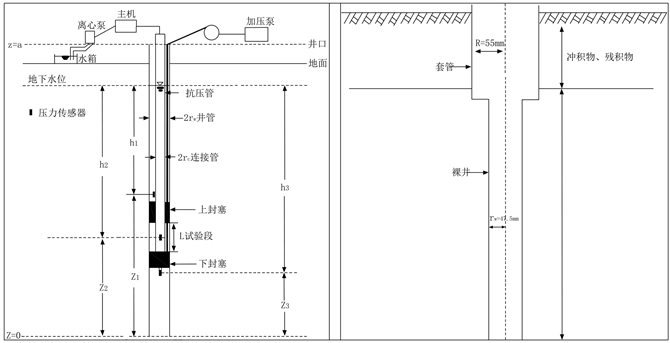Figure 2. Schematic diagram of the double plug hydrogeological system and drilling diagram

3.3. 试验方法

3.4. 结果分析Table 1. List of micro water test data analysis results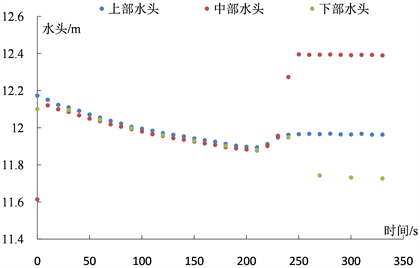Figure 3. Pressure duration curve of borehole 12 compression process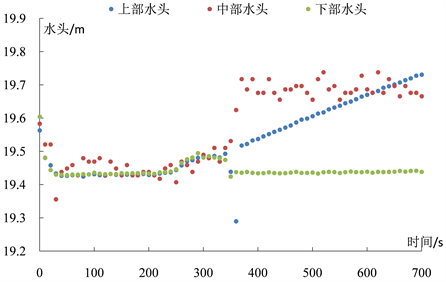Figure 4. Pressure duration curve of bore 13 compression process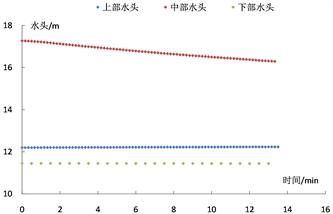Figure 5. 12-1 micro-water test pressure duration curve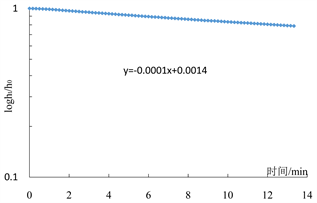Figure 6. Straight-line fitting of the Hvorslev model in section 12-1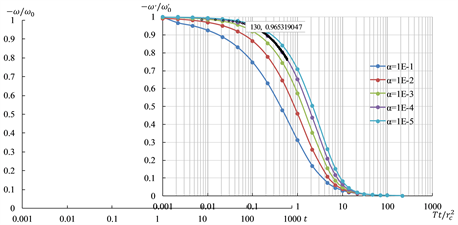Figure 7. Figure 12-1 CBP model fitting curve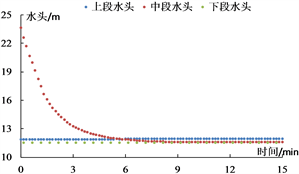Figure 8. 12-2 micro-water test pressure duration curve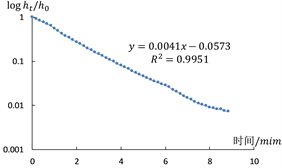Figure 9. Straight-line fitting of the Hvorslev model in section 12-2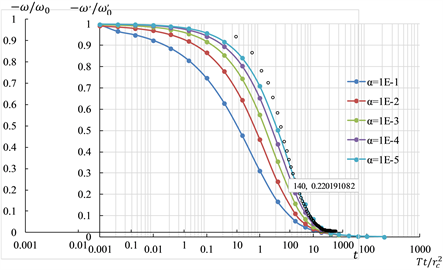Figure 10. 12-2 CBP model fitting curve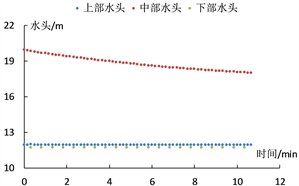Figure 11. 12-3 micro-water test pressure duration curve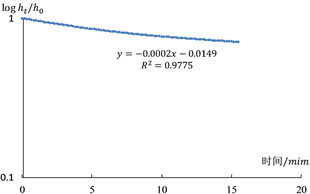Figure 12. Straight-line fitting of the Hvorslev model in section 12-3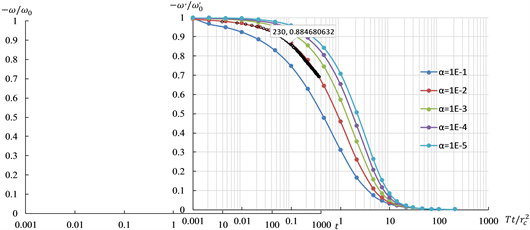Figure 13. Figure 12-3 CBP model fitting curve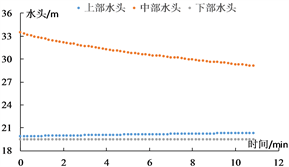Figure 14. 13-1 micro-water test pressure duration curve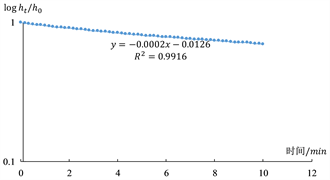Figure 15. Straight-line fitting of the Hvorslev model in section 13-1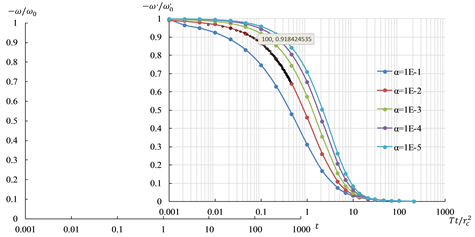Figure 16. Figure 13-1 CBP model fitting curveFigure 17. 13-2 micro-water test pressure duration curve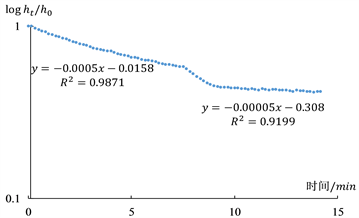Figure 18. Straight-line fitting of the Hvorslev model in section 13-2Figure 19. Figure 13-2 CBP model fitting curve

4. 结论

1) 对于同一段微水试验数据，CBP模型与Hvorslev模型求算的裂隙岩体渗透系数基本一致，与压水试验所得岩体渗透系数相比，结果偏大，这是由于微水试验影响范围较小，所求参数仅能反映钻孔附近有限范围内含水层的渗透特性，但该方法操作方便、成本低且计算简单，在快速定性获取裂隙介质中水文地质参数具有较好的应用前景。

2) 在低渗透性裂隙介质中开展微水试验时，钻孔水位的瞬时改变量宜大而不宜小，且应增大数据采集频率，多获取前期水位数据用于推算渗透系数。

3) CBP模型采用标准曲线配线法求参，可以求算含水介质的贮水系数，但其标准曲线具有一定的相似性，曲线系数α对标准曲线的敏感性较差，其求得的含水层贮水系数精度不高。

4) 与传统压水试验对比，微水试验所求的渗透系数偏大，微水试验反映的是试验段钻孔附近局部岩体渗透特征，压水试验是反映一定范围内试验段整体渗透特性。

 McElwee, C.D. (2002) Improving the Analysis of Slug Tests. Journal of Hydrology, 269, 122-133.
https://doi.org/10.1016/S0022-1694(02)00214-7

 McElwee, C.D., Bohling, G.C. and Butler Jr., J.J. (1995) Sensitivity Analysis of Slug Tests. Part 1. The Slugged Well. Journal of Hydrology, 164, 53-67.
https://doi.org/10.1016/0022-1694(94)02568-V

 McElwee, C.D., Butler Jr., J.J., Bohling, G.C. and Liu, W. (1995) Sensi-tivity Analysis of Slug Tests. Part 2. Observation Wells. Journal of Hydrology, 164, 69-87.
https://doi.org/10.1016/0022-1694(94)02569-W

 张昌新. 基于Hvorslev模型的微水试验应用[J]. 铁道勘察, 2016, 42(2): 16-20.

 黄先伍, 唐平, 缪协兴, 等. 破碎砂岩渗透特性与孔隙率关系的试验研究[J]. 岩土力学, 2005, 26(9): 1385-1388.

 Bird, R.B., Stewart, W.E. and Lightfoot, E.N. (2002) Transport Phenomena. 2nd Edition, John Wiley and Sons, Inc., New York.

 Kipp Jr., K.L. (1985) Type Curve Analysis of Inertial Effects in the Response of a Well to a Slug Test. Water Re-sources Research, 21, 1397-1408.
https://doi.org/10.1029/WR021i009p01397

 Cooper Jr., H.H., Bredehoeft, J.D. and Papado-poulos, I.S. (1966) Response of a Finite-Diameter Well to an Instantaneous Charge of Water. Water Resources Research, 3, 263-269.

 陈则连, 原国红, 赵丙君. 微水试验技术的应用研究[J]. 工程勘察, 2009, 37(7): 31-34.

Top